# Texas Go Math Grade 8 Lesson 4.4 Answer Key Proportional and Nonproportional Situations

Refer to our Texas Go Math Grade 8 Answer Key Pdf to score good marks in the exams. Test yourself by practicing the problems from Texas Go Math Grade 8 Lesson 4.4 Answer Key Proportional and Nonproportional Situations.

## Texas Go Math Grade 8 Lesson 4.4 Answer Key Proportional and Nonproportional Situations

Essential Question
How can you distinguish between proportional and nonproportional situations?

Determine if each of the following graphs represent a proportional or nonproportional relationship.

Question 1.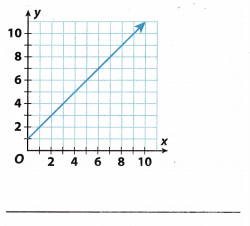The starting point of the graph is (0, 2) which means that it does not pass through the origin. Some functions will have a proportional relationship if the line passes through the origin, but it does not. So, this graph represents the nonproportional relationship.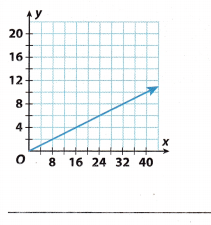Proportional relationship It does pass through the origin

Reflect

Question 3.
Communicate Mathematical Ideas In a proportional relationship the ratio $$\frac{y}{x}$$ is constant. Show that this ratio is not constant for the equation y = a – 14.
y = a – 14 Given
y = 16 – 14 = 2 Find a few values of y by substituting different values of a
y = 17 – 14 = 3
y = 18 – 14 = 4
$$\frac{2}{16}$$ = 0.125 Find the ratio $$\frac{y}{x}$$, where x represents a
$$\frac{3}{17}$$ = 0.176
$$\frac{4}{18}$$ = 0.222
The ratio is not constant.

Go Math 8th Grade Lesson 4.4 Proportional or Non-Proportional Question 4.
What If? Suppose another equation represents Keith’s age in months y given his age in years a. Is this relationship proportional? Explain.
y = 12a Since there are 12 months in a year

y = 12(1) = 12 Find a few values of y by substituting different values of a
y = 12(2) = 24
y = 12(3) = 36
$$\frac{12}{1}$$ = 12 Find the ratio $$\frac{y}{x}$$, where x represents a
$$\frac{24}{2}$$ = 12
$$\frac{36}{3}$$ = 12
Since the ratio is constant, the relationship is proportional.

Determine if each of the following equations represents a proportional or nonproportional relationship.

Question 5.
d = 65t
d = 65t Comparing with the linear equation
y = mx + b
Proportional relationship Since y-intercept b = 0

Question 6.
p = 0.1s + 2000
P = 0.1s + 2000 Comparing with linear relationship y = mx + b
This is a Non-Proportional relationship Since y-intercept b is not equal to 0
This is a Non-Proportional Relationship

n = 450 – 3p
n = 450 – 3p Comparing with the linear equation y = mx + b
Non-proportional relationship Since y-intercept b = 450

Question 8.
36 = 12d
36 = 12d Comparing with the linear equation y = mx + b
Non-proportional relationship The graph will not pass through the origin. It is a horizontal line.

Determine if the linear relationship represented by each table is a proportional or nonproportional relationship.

Question 9.To know if some linear relationship is proportional or nonproportional, we need to use ratio $$\frac{y}{x}$$, which needs to be constant for proportionality.
From the given table, we have values for x and y, so just put them into the calculation.
$$\frac{y}{x}$$ = $$\frac{30}{2}$$ = 15
= $$\frac{90}{8}$$ = 11.25
= $$\frac{150}{14}$$ = 10.71
Thus, the obtained values are not constant so this Linear relationship is not proportional.

Lesson 4.4 Proportional and Non-Proportional Relationships Question 10.$$\frac{1}{5}$$ = 0.2 Find the ratio $$\frac{y}{x}$$
$$\frac{8}{40}$$ = 0.2
$$\frac{13}{65}$$ = 0.2
The ratio is constant, hence the relationship is proportional.

Question 11.
Compare and contrast the following two situations.c = 20h Test-Prep Center A

The cost of attending Test-Prep Center A is proportional. The hourly rate is $20 which is less than Test-Prep Center B. Comparing with the linear equation y = mx + b where b = O C = 25h – 100 Test-Prep Center B where h is the number of hours and 1oo is a coupon The cost of attending Test-Prep Center B is non-proportional. The hourly rate is$25. It offers a coupon for an initial credit but its hourly rate is higher. Center B will cost more in the long run. Comparing with the Linear equation y = mx + b

Determine if each relationship is a proportional or nonproportional situation. Explain your reasoning. (Example 1, Example 2, Example 4)

Question 1.Look at the origin.
From the graph, we can see that the line is passing through the origin. When some linear function is passing
through the origin its relationship is proportional, and the y-intercept (‘b”) is equal to 0.

Question 2.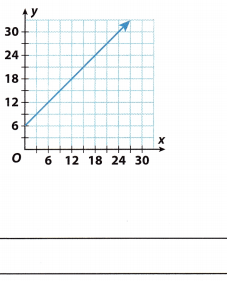From the graph, we can see that the line is not passing through the origin. When some linear function is not passing through the origin its relationship is nonproportional, and the y-intercept (“b”) is b ≠ 0.

Lesson 4 Skills Practice Proportional and Non-Proportional Relationships Answer Key Question 3.
q = 2p + $$\frac{1}{2}$$
Compare the equation with y = mx + b
q = 2p + $$\frac{1}{2}$$
The equation is in the form y = mx + b, with p being used as the variable instead of x and q instead of y. The value of in is 2, and the value of b is $$\frac{1}{2}$$. Since b is not 0, the relationship presented through the above equation is non-proportional.

Non-proportional

Question 4.
v = $$\frac{1}{10}$$u
To determine if the relationship of function is proportional or nonproportional, let us compare the equation in
slope-intercept form. After comparing the equations, determine the y-intercept b, if b = 0, then the relationship is
proportional, if b ≠ 0, then it is a non-proportional relationship.

Given the equation v = $$\frac{1}{10}$$u, it is a pattern of y = mx + b, where variable y is being used as y and u as r. The value of m is $$\frac{1}{10}$$, and the value of b = 0.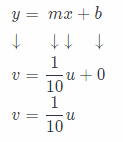Since the value of b is equal to 0, then the relationship between u and v is proportional.
See the explanation.

The tables represent linear relationships. Determine if each relationship is a proportional or nonproportional situation. (Example 3, Example 4)

Question 5.Find the quotient of y and x.
To know if some linear relationship is proportional or nonproportional, we need to use ratio $$\frac{y}{x}$$, which needs to be constant for proportionality.

From the given table, we have values for x and y, so just put them into my calculation
\begin(aligned}
\dfrac{y}(x}&=\dfrac{12}{3)=4\
&=\dfrac{36}{91}=4\
&=\dfrac{84}{21}=4
\end{atigned}
Thus, the obtained values are constant so this linear relationship is proportional.$$\frac{4}{22}$$ = $$\frac{2}{11}$$ Find the ratio $$\frac{y}{x}$$
$$\frac{8}{46}$$ = $$\frac{4}{23}$$
$$\frac{10}{58}$$ = $$\frac{5}{29}$$
Since the ratio is not constant, the relationship is non-proportional.

Question 7.
The values in the table represent the number of households that watched three TV shows and the ratings of the shows. The relationship is linear. Describe the relationship in another way. (Example 4)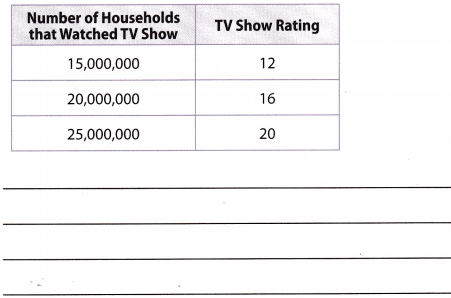The number of households that watched TV shows represents the æ and the TV show rating represents y. We know that the relationship is Linear, but we need to find out if the linear relationship is proportional or nonproportional.
To know if some linear relationship is proportional or nonproportional, we need to use ratio $$\frac{y}{x}$$, which needs to be constant for proportionality.
Given values of x and y, put into the calculationThus, the obtained values are constant, so this linear relationship is proportional.

Essential Question Check-In

Question 8.
How are using graphs, equations, and tables similar when distinguishing between proportional and nonproportional linear relationships?
The ratio between y to x is constant when the relationship is proportional. Graphs, tables, and equations all can be used to find the ratio. The ratio is not constant when the relationship is non-proportional.

Proportional and Non-Proportional Lesson 4.4 Answer Key Question 9.
The graph shows the weight of a cross-country team’s beverage cooler based on how much sports drink it contains.a. Is the relationship proportional or nonproportional? Explain.
Non-proportional
The graph does not pass through the origin. The graph of a proportional relationship must pass through the origin

b. Identify and interpret the slope and the y-intercept.
Slope = $$\frac{12-10}{4-0}$$ = 0.5 Finding the slope using any two given points by Slope(m) = (y2 – y1) ÷ (x2 – x1) where (x2, y2) = (4, 12)
(x1, y1) = (0, 10)
The slope shows that each cup of sports drink weighs $0.5 lb. y-intercept = 10 From the graph when x = 0 the y-intercept is the weight of the empty cooler, which is 10 lbs For 10-11, tell if the relationship between a rider’s height above the first floor and the time since the rider stepped on the elevator or escalator is proportional or nonproportional. Explain.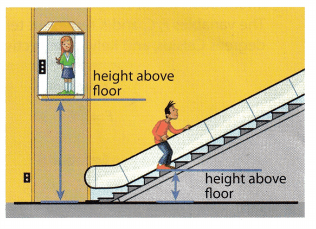Question 10. The elevator paused for 10 seconds after you stepped on before beginning to rise at a constant rate of 8 feet per second. Answer: The rider’s height above the first floor represents the y-axis and the time since the rider stepped on the elevator or escalator represents the x-axis. From the moment the rider steps on the elevator, the distance traveled at a certain time is counted. Only after 10 seconds, the elevator did start moving. For the first 10 seconds, the elevator did not move at all there wasn’t any shift in that time. If we tried to show this with a graph, the first point would be (10, 0), which means that the graph would start to rise constantly only with the starting point 10 on the x-axis. If this is the starting point and not (0, 0), i.e. the origin, this linear relationship is not proportional. Go Math Lesson 4.4 8th Grade Practice Answer Key Question 11. Your height, h, in feet above the first floor on the escalator is given by h = 0.75t, where t is the time in seconds. Answer: The given equation h = 0.75t is the form of the equation y = mx + b, where h is the value instead of y, and t is the value instead of x. In the given equation there is no y-intercept (b), thus our b is equal to 0, b = 0. Some linear relationships are proportional if the y-intercept is equal to 0, so the given linear relationship is proportional. Question 12. Analyze Relationships Compare and contrast the two graphs.Answer: Graph A represents a linear relationship while Graph B represents a exponential relationship. They both pass through the origin and the value of y increases with an increase in x. Question 13. Represent Real-World Problems Describe a real-world situation where the relationship is linear and nonproportional. Answer: The dentist receives a monthly fee of 20 for each patient and an additional 5 for each patient’s arrival. The equation is y = 5x + 20, where y represents total monthly income and x represents the number of patients admitted. The 20 fee represents the y – intercept(b). This relationship is linear but it is not proportional because the value of y-intercept is bigger than 0. i.e.b\not=0$.

Texas Go Math Grade 8 Lesson 4.4 H.O.T. Focus On Higher Order Thinking Answer Key

Question 14.
Mathematical Reasoning Suppose you know the slope of a linear relationship and one of the points that its graph passes through. How can you determine if the relationship is proportional or nonproportional?
Use the graph and the given point to determine the second point. Connect the two points by a straight line. If the
graph passes through the origin, the relationship is proportional and if the graph does not pass through the origin, the relationship is non-proportional.

Texas Go Math Grade 8 Pdf Practice and Homework Lesson 4.4 Question 15.
Multiple Representations An entrant at a science fair has included information about temperature conversion in various forms, as shown. The variables F, C, and K represent temperatures in degrees Fahrenheit, degrees Celsius, and Kelvin, respectively.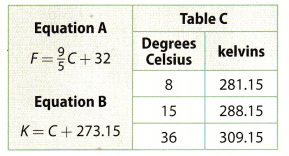a. Is the relationship between kelvins and degrees Celsius proportional? Justify your answer in two different ways.
1st – Equation B, K = C + 273.15, includes the values of temperatures in kelvins and degrees Celsius. This
equation is the form of the equation y = mx + b. The value of y-intercept (b) in equation B is bigger than 0, and
when b ≠ 0 the linear relationship is not proportional.

2nd – To know if some linear relationship is proportional or nonproportional, we need to use ratio $$\frac{y}{x}$$, which needs to be constant for proportionality. From the given table plot the values of x (degrees Celsius) and y (kelvin) into the calculation.
y = $$\frac{281.15}{8}$$ = 35.14
= $$\frac{288.15}{15}$$ = 19.21
= $$\frac{309.15}{36}$$ = 8.59
From the obtained values we see that they are not constant which means that the given equation is not proportional

b. Is the relationship between degrees Celsius and degrees Fahrenheit proportional? Why or why not?